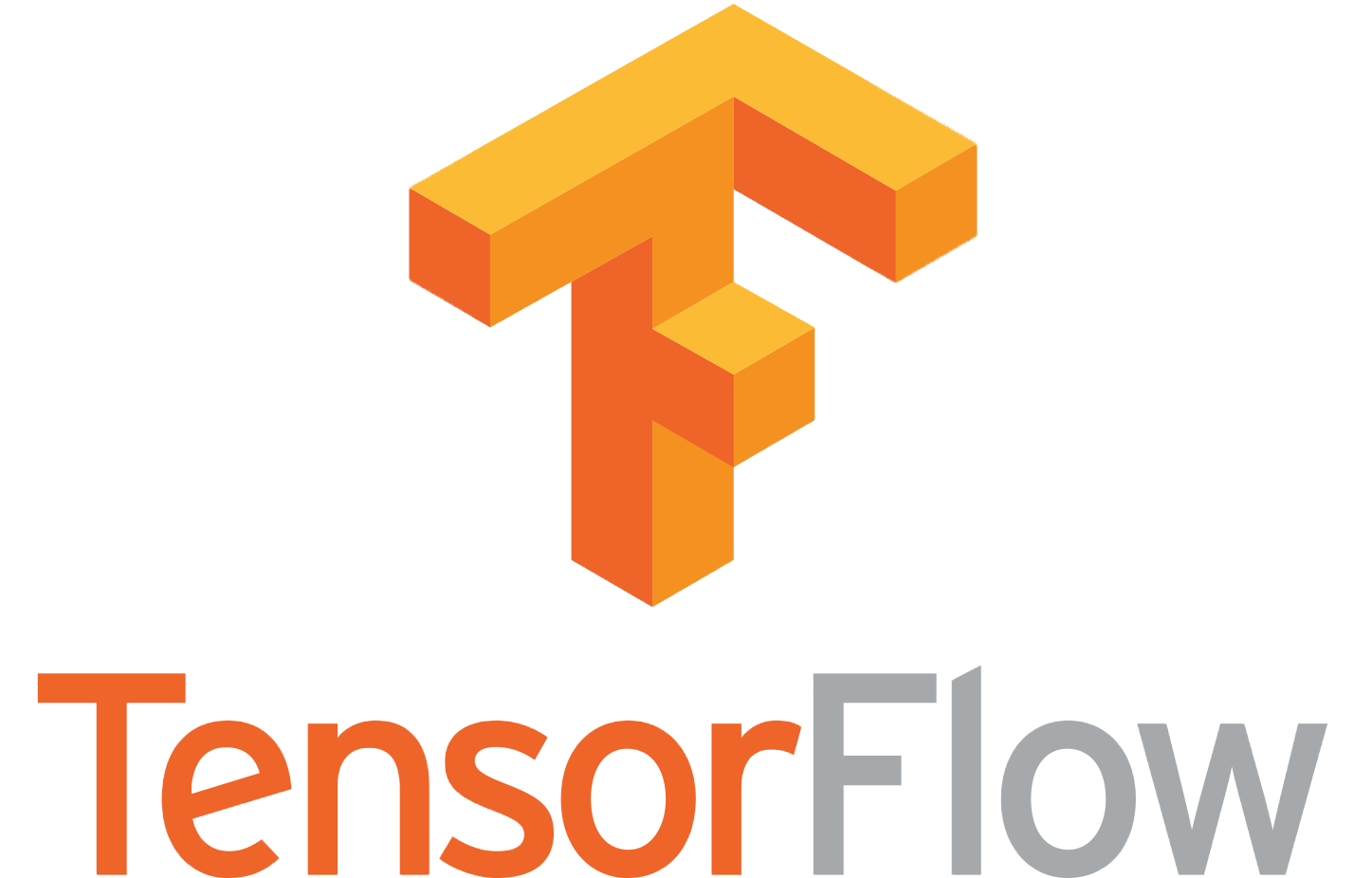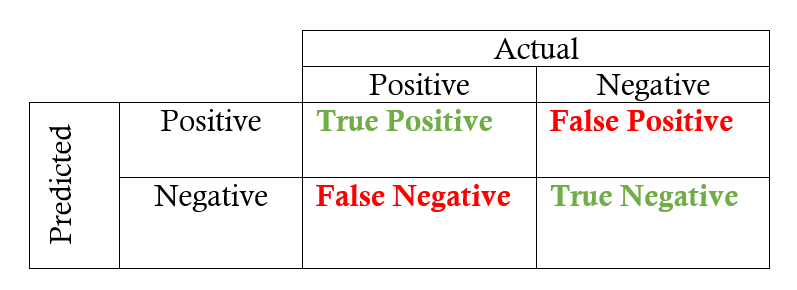TensorFlow cheat sheet
Machine Learning frameworkprint

TensorFlow version: 1.x - Date: Octobre 2018

# Introduction

TensorFlow is a Machine Learning framework developed by Google Inc. It includes different binding : C++, Java, Golang, Python, Javascript ... Python API is the most used by developpers

# Import

``````import tensorflow as tf
``````

# Module tf

Bring in all of the public TensorFlow interface.

``````#Classes:

tf.Graph() '''A TensorFlow computation, represented as a dataflow graph.'''

tf.device() '''A TensorFlow class to define the device (cpu/gpu) to use '''

tf.Operation() '''Represents a graph node that performs computation on tensors.'''

tf.Session()'''A class for running TensorFlow operations.'''

#Functions:

'''Returns x + y element-wise.'''

'''Return a tensor with the same shape and contents as input. (name: A name for the operation (optional))'''
b = tf.identity(a, name=None)

'''Returns the index with the largest value across axes of a tensor
example: for a 2D tensor, axis=1 refers to rows'''
c = tf.argmax(input, axis=None,name=None)

'''Computes square root of x element-wise.'''
d = tf.sqrt(x, name=None)
``````

More functions are available on the following link : https://www.tensorflow.org/api_docs/python/tf

# Module tf.data

This module contains different class used to manipulate data. Different files format are supported. It also introduces special classes fitted to TensorFlow for encoding/decoding data.

``````# Load the training data into two NumPy arrays, for example using `np.load()`.
features = data["features"]
labels = data["labels"]

# Assume that each row of `features` corresponds to the same row as `labels`.
assert features.shape == labels.shape

dataset = tf.data.Dataset.from_tensor_slices((features, labels))
``````

If you choose tfrecord format for encoding your data, you can use the following way to read (parse) your file(s):

``````# It accepts one or more filenames.
filenames = ["/var/data/file1.tfrecord", "/var/data/file2.tfrecord"]
dataset = tf.data.TFRecordDataset(filenames)
#Apply a transformation function to your data
dataset = dataset.map(func)
``````

If your data is contained in text files, you can use the following way to read (parse) it:

``````# It accepts one or more filenames.
filenames = ["/var/data/file1.txt", "/var/data/file2.txt"]
dataset = tf.data.TextLineDataset(filenames)
``````

In order to iterate over the dataset, TensorFlow provides the Iterator class:

``````# The returned iterator will be in an uninitialized state, and you must run the iterator.initializer operation before using it:
iterator = dataset.make_initializable_iterator()
tf.Session().run(iterator.initializer)
#Or use one_shot iterator that will be automatically initialized:
iterator = dataset.make_one_shot_iterator()
``````

# Module tf.nn

This module introduces basic functions for computer vision and sequential data (ex : text)

``````# Build a graph:
input_img = tf.placeholder(tf.float32, [batch_size, height, width, channels])
filter_img = tf.Variable(tf.random_uniform([7,7,3,target_channels]))

#strides: , padding can take the values "SAME" to maintain  the same dimension of the input or "VALID"
net = tf.nn.conv2d(input_img, filter_img, strides=[1,2,2,1], padding="SAME")
``````

More functions are available on the following link : https://www.tensorflow.org/api_docs/python/tf/nn

# Module tf.metrics

In order to evaluate your model, TensorFlow provides you with different functions that you can call during the computation graph. For each target response, we compare it with the value predicted by the model:

``````accuracy = tf.metrics.accuracy(labels,predictions)
``````

Calculates how often predictions matches labels. The accuracy function creates two local variables, total and count that are used to compute the frequency with which predictions matches labels. This frequency is ultimately returned as accuracy: an idempotent operation that simply divides total by count.``````precision = tf.metrics.precision(labels,predictions)
``````
``````recall = tf.metrics.recall(labels,predictions)
``````

Most of the functions provided can be applied for a classification task More functions are available on the following link : https://www.tensorflow.org/api_docs/python/tf/metrics

# Module tf.train

This module introduces basic classes and functions to monitor your training. In order to train your model, you need to use an optimizer that will handle the backpropagation algorithm. TensorFlow provides many implemantations of famous optimizers:

``````#We suppose that we have defined the loss function
your_learning_rate = value
learning_rate = your_learning_rate
)

#Define the training operation:
train_op = optimizer.minimize(loss)
``````

Below a list of the different optimizers, that you can find in the following link: https://www.tensorflow.org/api_docs/python/tf/train

``````tf.train.AdadeltaOptimizer #M. D. Zeiler
``````tf.train.MonitoredSession